﻿ The Momentum of New Matter Replaces Dark Energy and Explains the Expansion of the UniversePublications are Open
Access in this journal
Article Versions
Export Article
• Normal Style
• MLA Style
• APA Style
• Chicago Style
Research Article
Open Access Peer-reviewed

The Momentum of New Matter Replaces Dark Energy and Explains the Expansion of the Universe

Jarl-Thure Eriksson
International Journal of Physics. 2018, 6(5), 161-165. DOI: 10.12691/ijp-6-5-4
Received October 03, 2018; Revised November 06, 2018; Accepted November 19, 2018

Abstract

A new formulation of the Friedmann-Lemaître-Robertson-Walker equation has been developed in a previous paper. The present study analyses an alternative approach to the cosmological constant Λ from the perspective of the so called CBU model (Continuously Breeding Universe). Instead of having a cosmological constant responsible for the expansion, an excess pressure is boosted by a bang factor β. It is assumed that expansion is caused by the continuous addition of matter, due to, say, electron-positron fluctuations. Even if only a fraction of virtual pairs stay alive, while the majority returns to nothingness, the phenomenon is vigorous enough to explain the expansion. The momentum change due to the inflow of new matter provides a force and accordingly a pressure explaining the expansion. An equation of said pressure is derived and then interpreted as the equivalent to the cosmological 'constant', Λeq. The momentum pressure provides a perfect match to the dark energy density.

1. Introduction

In a paper published 1917 Albert Einstein introduced the cosmological constant Λ, 1, the purpose of which was to remove the dynamic instability of the universe as described by the General Theory of Relativity. Later Einstein regretted the additional term as the observations by Edwin Hubble showed that the universe was expanding. In principle Einstein could have envisaged the expansion, but for the wrong reason.

In order to expand, the system needs a "driving force", which is the reason why the cosmological constant has been reintroduced. In the Standard Cosmological Model (ΛCDM) Λ represents the dark energy content, also considered as the vacuum energy.

However, Einstein's paper of 1917 is interesting from some other points of view. He derives an equation for the curvature radius not very far from the Standard radius of the observable universe. He also speculates about the geometry of a space turning into itself.

In a recent paper the present author discusses the consequences that emerge, when certain well-founded assumptions are applied to the Friedmann equation, 2. As a hypothesis the universe started from a quantum fluctuation, cf. Tryon, 3, and is expanding due a continuous creation of new matter, e.g. electron-positron fluctuations. The idea of continuous matter creation was first proposed by Fred Hoyle, 4, and Hermann Bondi & Thomas Gold, 5, in the so called steady state theory. An addition of matter would keep the energy density constant in spite of expansion. In 1974 Paul Dirac wrote: "One might assume that nucleons are created uniformly throughout space, and thus mainly in intergalactic space. We may call this additive creation", 6.

If matter and accordingly energy is increasing, there must be a balancing mechanism in the form of negative energy. Alan Guth, 7, and Lawrence Krauss, 8, have been proponents of the idea, that all the energy in the universe sum up to zero, negative energy is provided by potential gravitational energy.

The model developed in Ref. 2 is called the Continuously Breeding Universe, CBU. The universe emerges from an initial electron-positron fluctuation. Acceleration is extremely high at the beginning, but decreases asymptotically to a very modest value of gu = 1,066·10-11 m/s2 today. The model leads to a universe in good agreement with estimates based on satellite data. For instance, the age to proper length diagram, i.e. light-cone diagram, is in very good conformity with the Planck data of 2013. The result requires that the Hubble's constant is Ho = 68,24·103 m/s/Mpc, just at the upper limit of the most recent results. The density of ordinary matter is 4,54·10-28 kg/m3, just within the limits given by NASA, 9.

One important result of the CBU model is a solution to the dark matter problem, which originates from the unexpected measurement results of galactic rotational speeds. The discrepancies are accurately explained by a Coriolis effect due to the accelerated expansion.

In the present article we will discuss some issues in the Einstein 1917 paper. Using the Robertson-Walker metric a comprehensive derivation of the Friedmann acceleration equation is accomplished. The Cosmological constant Λ is replaced with a "bang-factor" β, it is shown that both alternatives are consistent with the original Friedmann-Lemaître-Robertson-Walker equation. Finally it is shown that dark energy is explained by the momentum pressure caused by the continuous addition of new matter.

2. Hypotheses

2.1. Continuous Matter Creation

Matter "created from the inside" is a logic explanation to the expansion of the universe. Several physicists have been proponents of this idea, as pointed out in the introduction. It is known for a fact that quantum fluctuations occurs constantly causing a quantum foam, cf. John Wheeler as quoted by Leonard Susskind, 10. From reasons we don't know yet some of those virtual particles have time to annihilate into radiation and are incapable of returning to "nothingness". As a result there is a net increase of energy.

Hypothesis 1: The universe expands due to the addition of new matter. The positive energy is balanced by an equal amount of negative energy in the form of potential gravitational energy as reflected by the increase of space.

2.2. Relation between Energy and Space

In the years right after the publication of the General Theory of Relativity many implications were debated, one of which seemed to be a weird coincidence. If one squared the ratio of the radius of the universe ru and the estimated radius of the proton rp, one would get a very large number corresponding to the ratio of the universe mass Mu and the proton mass mp: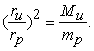(1)

Even if the motive to find a direct relation between the cosmos and the micro world was somewhat obscure, the fact that the energy of matter would be proportional to the radius squared was considered more than just a coincidence. This led Paul Dirac to define his Large Number Hypothesis (LNH) in 1937, 11.

Hypothesis 2: The total positive energy of the universe is proportional to the surface of a sphere having the radius ru. We define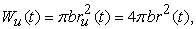(2)

where r = ru/2 is the radius of the observable universe, b is a universal constant to be determined in section 4.

2.3. Size Dependent Gravitational Constant

Mach's principle, after the Austrian physicist Ernst Mach, had a decisive impact on Einstein's thinking. According to the principle inertia is caused by the collective action of all masses in the universe. In 1953 Dennis Sciama, the mentor of Stephen Hawking, published a paper on the origin of inertia and concluded that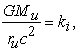(3)

where ki is very close to 1. Here we assume that ki = 1, which also has been suggested by Brans and Dicke in 1961, 12.

Assuming that the mass Mu includes the equivalent mass of radiation, we have that Mu = Wu/c2 = 4πbr2/c2 where Wu is the total positive energy. When this result is substituted into eq. (3), we obtain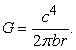(4)

Hypothesis 3: The gravitational constant G is inversely proportional to the radius of the observable universe.

This is one of the most controversial issues in the CBU model. Astrophysicists of today strongly argue in favor of a constant G. However, it is logical that the gravitational force varies with the curvature (which is related to the radius r). A variable G means that the Planck length lP is of the nuclear particle order in the early universe. An inflationary phase is not necessary.

3. Peculiarities in Einstein's Paper on the Cosmological Constant

According to the story Einstein regretted his 1917 paper, 1, by saying it was "his biggest blunder". It is uncertain if this was his real wording. He was discussing the paper with George Gamow, 13. Probably they were using the German language, so most certainly Einstein did not use the word "blunder".

The paper includes several interesting features, whatever Einstein's opinion was. He derives an equation for what he calls the curvature radius, which later has been referred to as the Einstein radius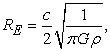(5)

where ρ is the average mass density of the universe.

If we assume that ki = 4/3, ru = 2RE and Mu=32π·ρ·RE3/3 and substitute them into eq (3), we will arrive at exactly the Einstein radius equation. Using the latest NASA estimate for the density of ordinary matter, ρ= 4,5·10-28 kg/m3, we obtain RE = 4,88·1026 m, a value only 11 % larger than the ΛCDM model radius of 4,396·1026 m.

The relevance of eq. (3) is emphasized by Einstein. Did he actually show that G is dependent of r (= RE)?

Another interesting detail is the remark "...als auch den Radius R des sphärischen Raumes und dessen Volumen 2π2R3" (...and also the radius R and the volume 2π2R3 of the spherical space). Einstein uses the equation for the hyper-surface S4 of a 4d space (cf. Wolfram MathWorld: Four-dimensional geometry), which also is the equation of the volume of a horn torus, i.e. a torus having the same major and minor radii (no central hole). The observer stands on the axis of revolution, the cross section is the observable universe, the horizon circumference is 2πR. The axis of revolution, length 2πR, forms the curved space.

The main purpose of Einstein's paper was the derivation of the cosmological constant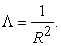(6)

The value appears to be circa 26 times smaller than the expansion requires, as we shall see in section 5.

At the end of the paper Einstein states "The term (Λ) is necessary only for the purpose of making possible a quasi-static distribution of matter, as required by the fact of the small velocities of the stars." An expanding universe was not on his agenda in 1917.

4. Key Parameters

At the initial moment a positron and an electron form the maiden universe. The "Eucledian" distance between the particles is 2ri, but through the curved space the interaction distance is πri. The energy equilibrium is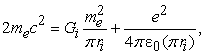(7)

where me and e are the electron mass and charge respectively. Gi is obtained from eq. (3) given that Mu = 2me, r = ri and k = 1. We solve ri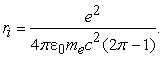(8)

The value is ri = 0,533381·10-15 m, a value of the same order of magnitude as the proton charge radius.

The energy constant of eq. (2) is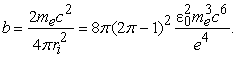(9)

The value is b = 0,458009·1017 J/m2.

From eq. (4) we are now able to determine the radius r of the observable universe today. The value is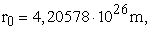slightly smaller than the official value.

By substituting b from eq. (9) into eq. (4) we obtain an expression for G consisting of physical constants and the radius r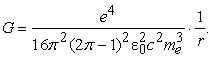(10)

5. Expansion According to the Robertson-Walker Metric

Einstein's field equation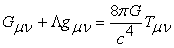(11)

has an analytic solution provided the energy-momentum tensor is isotropic and homogeneous. In the Robertson-Walker metric the solution takes the form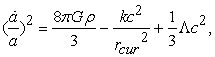(12)

where a is the scale factor, ρ is the average mass density (radiation included), k is the curvature factor, -1, 0 or 1, rcur is the curvature radius and Λ is the cosmological constant. If we assume that the universe is flat in space-time, k is zero.

Our next step is to derivate with respect to time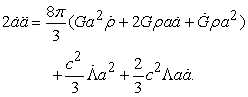(13)

We can now write(14)

The equation differs from the accustomed form by taking into account time changes in G and Λ.

In order to introduce the pressure responsible for the expansion we start with the 1. Law of Thermodynamics: dW + pdV = 0. We derivate with respect to time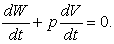(15)

The rate of volume expansion is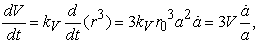(16)

where r = ar0 and V = kV·a3r03, the volume of the universe. kV is a volume factor, 32π/3 for a sphere and 2π2 for a 4d hyper-surface S4. Here it is irrelevant which one we use.

The change of work is obtained from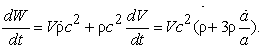(17)

When eqs. (16) and (17) are substituted into eq. (15), we obtain an expression containing p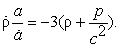(18)

When substituted into eq. (14) we get the complete version of the Friedmann-Lemaître-Robertson-Walker (FLRW) equation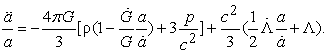(19)

From the relations W = πbru2 and dW = 2πbru·dru = -pdV, we derive p = - b/2ru = - b/4aro. Further from eqs. (2) and (4) follows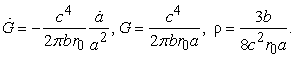These parameters as functions of the scale factor a are substituted into eq. (19). It appears that the first term vanishes, which is a sign of an unstable state and the relevance of the initial hypotheses of section 2. We are left with the following equation consisting only of Λ-terms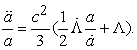(20)

We suggest the introduction of a new parameter β, which accounts for the additional pressure required to maintain the continuous expansion. This is an elegant way of dealing with the instability as compared to the cosmological constant, which is not considered a constant here. The extra pressure is provided by the continuous addition of matter. The alternative form of eq. (19) is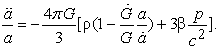(21)

When ρ, G and Ġ are substituted, the equation takes the form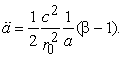(22)

The first time derivative of the scale factor a, i.e. expansion velocity, is solved by integration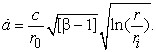(23)

This is the Hubble parameter h. A further integration of eq. (23) leads to a relation between the time and the size of the universe. The integration, which is presented in Ref. 2, comprises an error function solution of the form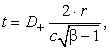(24)

where D+= D+(√ln(r/ri)) is the Dawson integral function. From eq. (24) we have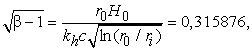(25)

where kh = 3,08567·1022 m/Mpc (H0 = h0kh). In Ref. 2 the Hubble parameter was estimated to H0 = 68,24 km/sMpc. We then have β=1,099778. The age of the present universe appears to be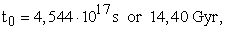slightly larger than the Standard model age of 13,8 Gyr. We have to remember though, that there is a substantial difference between the beginning phases, Big Bang plus inflation against a "slow" but strongly accelerating initial start.

If we substitute eq. (23) into the energy equation (12), we find the relation between β and Λ. Assuming that k = -1 and that rcur = r we have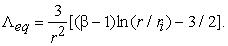(26)

The value of Λeq is 1,38·10-52 m-2.

If eq. (26) and its time derivative are substituted into eq. (20), we will end up with eq. (22) thereby showing the consistency between eqs (12) and (22).

6. Momentum Pressure Instead of Dark Energy

The force caused by the momentum of new matter is obtained from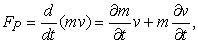(27)

where v is the velocity of expansion and m = 2meNe+e-. From eq. (1) follows that the number of fluctuations is Ne+e=(r/ri)2. Utilizing the relations given in eqs (9) and (23) we end up with an equation for the momentum force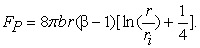(28)

The energy provided by the momentum force is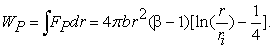(29)

In order to calculate the pressure pP we have to divide WP with the 4d hyper-surface S4 = 2π2r3. The momentum pressure is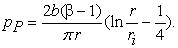(30)

In order to decide the nature of the curvature we change eq. (12) into a density equation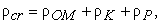(31)

where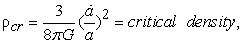(32)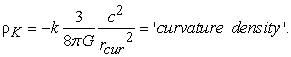(33)

The density ρP is obtained by dividing eq. (30) with c2. ρOM represents ordinary matter including radiation.

When the right hand side of eq. (31) is divided by ρcr we obtain an expression familiar from the ΛCDM model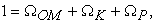(34)

where (obviously) ΩOM = ρOMcr, ΩK = ρKcr and ΩP = ρPcr.

Table 1 shows some characteristics of the CBU and the ΛCDM models, 14. When the CBU numbers are applied to eq. (34) and we further assume that rcur = r0 and k = -1, we end up with the following resultExpansion accelerates because of a 2,35 per mille overshot in the critical density

Hereby it is convincingly established that the universe expands hyperbolically and that the curvature radius of the 3d geometry of space is equal to the radius of the observable universe. It cannot be just a coincidence that the momentum pressure of eq. (30) perfectly balances the curvature and matter terms of eqs (31) and (34).

It must, however, be remembered that the introduction of β is an approximation, like Λ, and that a rigorous mathematical description of the early universe requires a deeper analysis of the origin of β and its time dependence.

Considering that no form of matching of the models has been performed the agreement between key characteristics in Table 1 is very good. The momentum pressure leads to a higher "dark energy" density, but eliminates the need for dark matter.

7. Conclusions

The CBU model has a sound foundation in physics. Results are in good agreement with the present description of the universe. The evolutionary route differs from the traditional FLRW t2/3-scenario, but fits experimental supernova data as far back in time as observations reach. In a previous paper, 2, it was shown that the acceleration of the expansion explains kinematic discrepancies in galaxies due to the Coriolis effect, dark matter is not required. This means that the space within a galaxy also expands, a condition questioned by some astrophysicists. Empty space should not be mixed with an ether, it is not a substance.

Vacuum fluctuations are known as a fact. It is reasonable to regard the foam maintained by the fluctuations of virtual particles and antiparticles as the breeding source of new matter. According to the CBU model space is directly connected to the energy content, an increase of matter/energy results in an increase in size and the over all curvature radius. Space "bends" throughout due to the changing curvature. However, local concentrations of matter cause distortions, very small in comparison to the over all curvature radius.

It is shown that the cosmological 'constant' can be replaced by a factor β describing the "inflow" of matter and thereby the primary origin of expansion. It seems more logical to presume a constant kick (β) to the expansion pressure than to postulate a size and time independent cosmological constant. The replacement leads to a completely new picture of the universe's past. The CBU theory provides a continuous mathematical description of the emerging universe. Decaying acceleration of expansion, decreasing G and a changing radius of curvature are factors making the interpretation of distant observations very complex.

The main finding of this paper is that dark energy has to be replaced by a kinetic energy constituent, the origin of which is the momentum of emerging matter. Equilibrium requires that space is hyperbolically curved and that the curvature radius equals the radius of the observable universe. There are no alternative solutions to eq. (12). This is a plausible result. In his 1917 paper Einstein intuitively presumed that r = rcur.

There are several open questions still to be answered. One concerns the mechanism responsible for the continuous breeding of new baryonic matter, the chain from positron-electron pairs to hydrogen atoms. Other questions are, how does the CMB fit into the model, what impact will an extremely high value of G in the past have on the redshift z? The answers may offer new avenues to explain the connection between gravity and quantum mechanics.

Summary of basic characteristics:

• The Universe is a complex emerging physical system.

• The Universe was initiated by a quantum fluctuation of an electrically charged elementary particle and its antiparticle.

• The Universe expands due to a continuous generation of elementary particle pairs.

• The positive energy of matter and radiation is the origin of space, outside the Universe there is no space, nothing.

• The positive energy of matter and radiation is balanced by an equal amount of negative potential gravitational energy.

• The space is curved into itself, the curvature radius is directly proportional to the size of the Universe.

• Stellar processes have no impact on the macroscopic expansion progress of the Universe.

References

  Einstein, A., Kosmologische Betrachtungen zur allgemeinen Relativitätstheorie. Sitzungsberichte der Preussischen Akad. d. Wissenschaften, 1917. In article  Eriksson, J.-T., A modified model of the universe shows how acceleration changes galaxy dynamics, International Journal of Physics Vol 6, No. 2, pp. 38-46, 2018. In article  Tryon, E. P., Is the universe a vacuum fluctuation?. Nature 247, pp. 396-397, 1973. In article View Article  Hoyle, F., A new model for the expanding universe, Mon. Not. Roy. Ast. Soc., 108, pp. 372-382, 1948. In article View Article  Bondi, H., Gold, T., The steady-state theory of the expanding universe, Mon. Not. Roy. Ast. Soc., 108, pp. 252-270, 1948. In article View Article  Dirac, P., Cosmological models and the Large Number hypothesis, Proc. R. Soc. Lond. A. 338, pp. 439-446, 1974. In article View Article  Guth, A. The Inflationary Universe: The quest for a new theory of cosmic origins, Perseus Books, 1997. In article  Krauss, L., Universe from nothing, Simon & Schuster, NY, 2012. In article  NASA, Aeronautics and Space Administration, Universe 101, 2014. In article  Susskind, L.,The Blach Hole War, Back Bay Books, NY, p.146, 2008. In article  Dirac, P. A. M., The cosmological constants, Nature 139, 323, 1937. In article View Article  Brans, C., Dicke, R. H., Mach's principle and a relativistic theory of gravitation, Physical Review, 124, 3, pp. 925-935, 1961. In article View Article  Weinstein, G., George Gamow and Albert Einstein: Did Einstein say the cosmological constant was the “biggest blunder” he ever made in his life?, arXiv:1310.1033v1 [physics.hist-ph], 2013. In article  Ade, P. A. R. et al. (Planck Collaboration), Planck 2015 results. XIII. Cosmological parameters, arXiv:1502.01589v3 [astro-ph.CO], 17 Jun 2016. In articleThis work is licensed under a Creative Commons Attribution 4.0 International License. To view a copy of this license, visit http://creativecommons.org/licenses/by/4.0/